# Confidence interval facts for kids

Kids Encyclopedia Facts

In statistics a confidence interval is a special form of estimating a certain parameter. With this method, a whole interval of acceptable values for the parameter is given instead of a single value, together with a likelihood that the real (unknown) value of the parameter will be in the interval. The confidence interval is based on the observations from a sample, and hence differs from sample to sample. The likelihood that the parameter will be in the interval is called confidence level. Very often, this is given as a percentage. The confidence interval is always given together with the confidence level. People may speak about the "95% confidence interval". The end points of the confidence interval are referred to as confidence limits. For a given estimation procedure in a given situation, the higher the confidence level, the wider the confidence interval will be.

The calculation of a confidence interval generally requires assumptions about the nature of the estimation process – it is primarily a parametric method. One common assumption is that the distribution of the population from which the sample came is normal. As such, confidence intervals as discussed below are not robust statistics, though changes can be made to add robustness.

## Meaning of the term "confidence"

The term confidence has a similar meaning in statistics, as in common use. In common usage, a claim to 95% confidence in something is normally taken as indicating virtual certainty. In statistics, a claim to 95% confidence simply means that the researcher has seen one possible interval from a large number of possible ones, from which nineteen out of twenty intervals contain the true value of the parameter.

## Practical example

A machine fills cups with margarine. For the example, the machine is adjusted so that the content of the cups is 250g of margarine. As the machine cannot fill every cup with exactly 250g, the content added to individual cups shows some variation, and is considered a random variable X. This variation is assumed to be normally distributed around the desired average of 250g, with a standard deviation of 2.5g. To determine if the machine is adequately calibrated, a sample of n = 25 cups of margarine is chosen at random and the cups are weighed. The weights of margarine are X1, ..., X25, a random sample from X.

To get an impression of the expectation μ, it is sufficient to give an estimate. The appropriate estimator is the sample mean: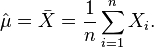$\hat \mu=\bar X = \frac{1}{n}\sum_{i=1}^n X_i.$

The sample shows actual weights x1, ...,x25, with mean: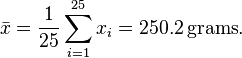$\bar x=\frac {1}{25} \sum_{i=1}^{25} x_i = 250.2\,\text{grams}.$

If we take another sample of 25 cups, we could easily expect to find values like 250.4 or 251.1 grams. A sample mean value of 280 grams however would be extremely rare if the mean content of the cups is in fact close to 250g. There is a whole interval around the observed value 250.2 of the sample mean within which, if the whole population mean actually takes a value in this range, the observed data would not be considered particularly unusual. Such an interval is called a confidence interval for the parameter μ. How do we calculate such an interval? The endpoints of the interval have to be calculated from the sample, so they are statistics, functions of the sample X1, ..., X25 and hence random variables themselves.

In our case we may determine the endpoints by considering that the sample mean X from a normally distributed sample is also normally distributed, with the same expectation μ, but with standard error σ/√n = 0.5 (grams). By standardizing we get a random variable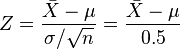$Z = \frac {\bar X-\mu}{\sigma/\sqrt{n}} =\frac {\bar X-\mu}{0.5}$

dependent on the parameter μ to be estimated, but with a standard normal distribution independent of the parameter μ. Hence it is possible to find numbers −z and z, independent of μ, where Z lies in between with probability 1 − α, a measure of how confident we want to be. We take 1 - α = 0.95. So we have: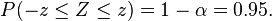$P(-z\le Z\le z) = 1-\alpha = 0.95. \,$

The number z follows from the cumulative distribution function: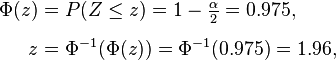\begin{align} \Phi(z) & = P(Z \le z) = 1 - \tfrac{\alpha}2 = 0.975,\\[6pt] z & = \Phi^{-1}(\Phi(z)) = \Phi^{-1}(0.975) = 1.96, \end{align}

and we get: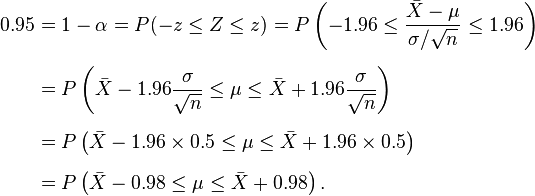\begin{align} 0.95 & = 1-\alpha=P(-z \le Z \le z)=P \left(-1.96 \le \frac {\bar X-\mu}{\sigma/\sqrt{n}} \le 1.96 \right) \\[6pt] & = P \left( \bar X - 1.96 \frac{\sigma}{\sqrt{n}} \le \mu \le \bar X + 1.96 \frac{\sigma}{\sqrt{n}}\right) \\[6pt] & = P\left(\bar X - 1.96 \times 0.5 \le \mu \le \bar X + 1.96 \times 0.5\right) \\[6pt] & = P \left( \bar X - 0.98 \le \mu \le \bar X + 0.98 \right). \end{align}

This might be interpreted as: with probability 0.95 we will find a confidence interval in which we will meet the parameter μ between the stochastic endpoints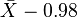$\bar X - 0{.}98 \,$

and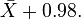$\bar X + 0.98. \,$

This does not mean that there is 0.95 probability of meeting the parameter μ in the calculated interval. Every time the measurements are repeated, there will be another value for the mean X of the sample. In 95% of the cases μ will be between the endpoints calculated from this mean, but in 5% of the cases it will not be. The actual confidence interval is calculated by entering the measured weights in the formula. Our 0.95 confidence interval becomes: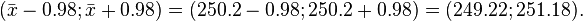$(\bar x - 0.98;\bar x + 0.98) = (250.2 - 0.98; 250.2 + 0.98) = (249.22; 251.18).\,$

As the desired value 250 of μ is within the resulted confidence interval, there is no reason to believe the machine is wrongly calibrated.

The calculated interval has fixed endpoints, where μ might be in between (or not). Thus this event has probability either 0 or 1. We cannot say: "with probability (1 − α) the parameter μ lies in the confidence interval." We only know that by repetition in 100(1 − α) % of the cases μ will be in the calculated interval. In 100α % of the cases however it does not. And unfortunately we do not know in which of the cases this happens. That's why we say: "with confidence level 100(1 − α) %, μ lies in the confidence interval."

The figure on the right shows 50 realisations of a confidence interval for a given population mean μ. If we randomly choose one realisation, the probability is 95% we end up having chosen an interval that contains the parameter; however we may be unlucky and have picked the wrong one. We'll never know; we are stuck with our interval.

## Images for kidsConfidence interval Facts for Kids. Kiddle Encyclopedia.